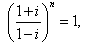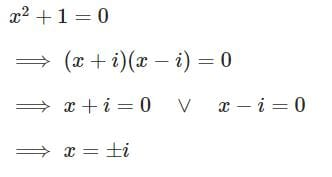## 10 Questions MCQ Test Mathematics For JEE | Test: Quadratic Equations

Description
Attempt Test: Quadratic Equations | 10 questions in 10 minutes | Mock test for JEE preparation | Free important questions MCQ to study Mathematics For JEE for JEE Exam | Download free PDF with solutions
QUESTION: 1

### Solve the quadratic equation x2 – ix + 6 = 0

Solution:

x2 - ix + 6 = 0
x2 - 3ix + 2ix - 6i2 = 0    { i2 = -1}
x(x-3i) + 2i(x-3i) = 0
(x+2i) (x-3i) = 0
x = -2i, 3i

QUESTION: 2

###so the least integral value of n is

Solution:

{(1 + i)/(1 - i)}n = 1
multiply (1 + i) numerator as well as denominator .
{(1 + i)(1 + i)/(1 - i)(1 + i)}n = 1
{(1 + i)²/(1² - (i)²)}n = 1
{(1 + i² +2i)/2 }n = 1
{(2i)/2}n = 1
{i}n = 1
we know, i4n = 1 where , n is an integer.
so, n = 4n where n is an integers
e.g n = 4 { because least positive integer 1 }
hence, n = 4

QUESTION: 3

### Solve the quadratic equation ix2 – 3x – 2i = 0

Solution:

x2−3x−2i=0,

or  x2 + 3ix − 2=0      (divided by i)
x= −3i ± [−9 + 4.1.2]1/2/2.1
= −3i ±  (−1)1/2/2
​= (−3i ± i)/2
=> x=−i or x=−2i

QUESTION: 4

Find the roots of the quadratic equation: x2 + 2x - 15 = 0?

Solution:

x2 + 5x - 3x - 15 = 0
x(x + 5) - 3(x + 5) = 0
(x - 3)(x + 5) = 0
⇒ x = 3 or x = -5.

QUESTION: 5

Solve the quadratic equation x2 +1 = 0

Solution:QUESTION: 6

The solution of the quadratic equation: 2x2 + 3ix + 2 = 0

Solution:

2x2 + 3ix + 2 = 0
we know, x = (-b ± √b2 - 4ac)/2a
x =  [-3i ± √(3i)2 - 4x2x2]/2x2
= -3i ± √-25/4
= i(-3±5)/4
x = i/2, -2i

QUESTION: 7

The solution of the quadratic equation : 2x2 – 4x + 3 = 0

Solution:

2x2 - 4x + 3 = 0
x = [-(-4) +- (√16-24)]/2(2)
x = (4 +- i√8)/4
x = (4 +- 2i√2)/(2 * √2 * √2)
x = 2(2 +- i√2)/(2 * √2 * √2)
x = 1 +- i/√2

QUESTION: 8

If one of the root of a quadratic equation with rational coefficients is rational, then other root must be

Solution:

Also, αβ = r/p, which is also rational. α + β = (a+√b) + (a-√b) = 2a, a rational number and, αβ = (a+√b)(a-√b) = a² - b, a rational number. So, the other root of a quadratic equation having the one root as (a+√b) is (a-√b), where a and b are rational numbers.

QUESTION: 9

If the sum of three consecutive terms of an increasing A.P. is 51 and the product of the first and third of these terms is 273, then the third term is

Solution:

Let these three terms are, a−d,aanda+d.
Then,
a−d+a+a+d=51⇒3a=51⇒a=17
Now, (a−d)(a+d)=273
⇒(17−d)(17+d)=273
⇒289−d2=273⇒d2=16
⇒d=4 (As d can not be negative.)
So, third term will be, 17+4=21.

QUESTION: 10

Solve the quadratic equation 9x2 + 16 = 0

Solution:

9x2 + 16 = 0
9x2 = -16
x2 = -16/9
x = ± 4/3 iUse Code STAYHOME200 and get INR 200 additional OFF Use Coupon Code